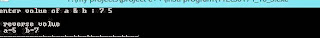## Sunday, 20 October 2013

### Basic question -5 (swapping values without using third variable )

How to Swap values of 2 variable without using third variable .
It is to simple If  we use third variable .

Let  variable are 'a' , 'b' , 'temp' . we have swap value  of variable 'a' & 'b'.  & 'temp' is third variable .

temp = a
a = b
b  = temp

Now solve it without using third variabe

a = a + b
b = a - b
a = a - b

#### C Program :

Without  Using third variable :

`````` #include<stdio.h>
void swap(int *p,int *q)
{          // operation to swap
*p=*p+*q;
*q=*p-*q;
*p=*p-*q;
printf(" \n reverse volue\n a=%d b=%d",*p,*q);
}
main()
{
int a,b;
printf("enter volue of a & b : ");
scanf("\n%d %d",&a,&b);
swap(&a,&b);
}
``````

#### OUTPUT :Using Third Variable :

`````` #include<stdio.h>
void swap(int *p,int *q)
{          // operation to swap
int temp;  // temp variabe
temp = *p;
*p = *q;
*q = temp;
printf(" \n reverse volue\n a=%d b=%d",*p,*q);
}
main()
{
int a,b;
printf("enter volue of a & b : ");
scanf("\n%d %d",&a,&b);
swap(&a,&b);
}
``````